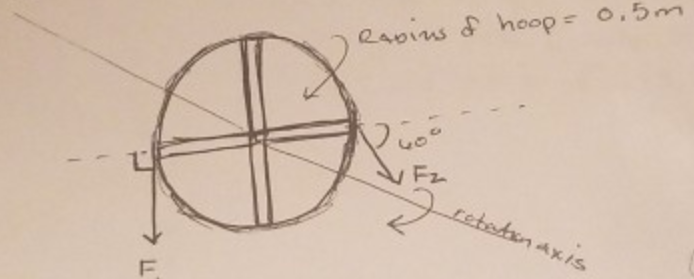# Problem: A bicycle tire is shown under the influence of 2 forces. The spokes of the tire are assumed to be long thin rods Each spoke has a mass of 2 kg. The tire is assumed to be a hoop or thin cylindrical shell of mass 10 kg. If F1 = 100 N and F2 = 100 N, calculate:The net torque, the moment of inertia, the angular acceleration, the # of revolutions, if the tire starts from rest, after 10 seconds.

###### FREE Expert Solution

In this problem, we'll list the concepts involved, then address them stepwise.

Torque:

$\overline{){\mathbf{\tau }}{\mathbf{=}}{\mathbf{r}}{\mathbf{F}}}$

Moment of inertia of a rod about the center:

95% (270 ratings)###### Problem Details

A bicycle tire is shown under the influence of 2 forces. The spokes of the tire are assumed to be long thin rods Each spoke has a mass of 2 kg. The tire is assumed to be a hoop or thin cylindrical shell of mass 10 kg.If F1 = 100 N and F2 = 100 N, calculate:

The net torque, the moment of inertia, the angular acceleration, the # of revolutions, if the tire starts from rest, after 10 seconds.